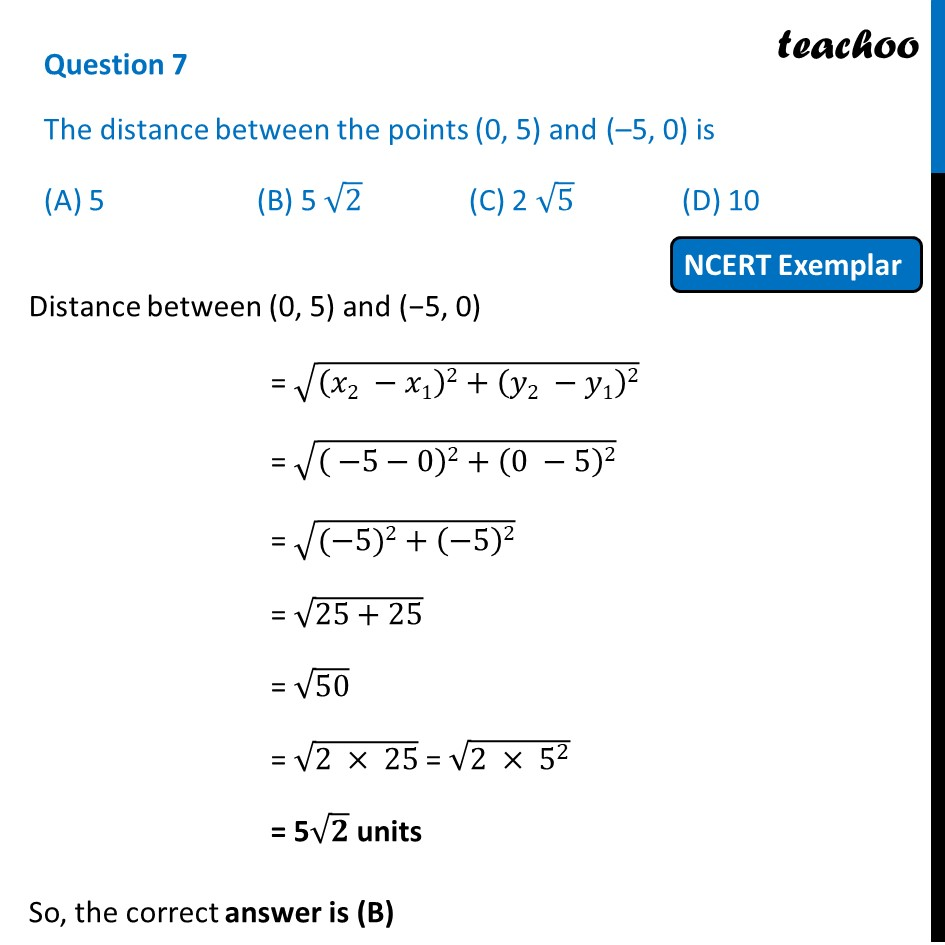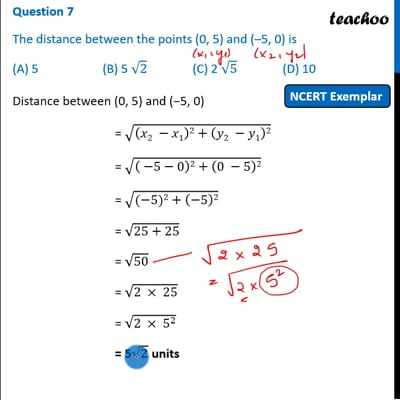NCERT Exemplar - MCQ

Chapter 7 Class 10 Coordinate Geometry
Serial order wise

## (A) 5   (B) 5 √2    (C) 2 √5    (D) 10This video is only available for Teachoo black users

Maths Crash Course - Live lectures + all videos + Real time Doubt solving!

### Transcript

Question 7 The distance between the points (0, 5) and (–5, 0) is (A) 5 (B) 5 √2 (C) 2 √5 (D) 10 Distance between (0, 5) and (−5, 0) = √((𝑥2 −𝑥1)2+(𝑦2 −𝑦1)2) = √(( −5−0)2+(0 −5)2) = √((−5)2+(−5)2) = √(25+25) = √50 = √(2 × 25) = √(2 × 5^2 ) = 5√𝟐 units So, the correct answer is (B)